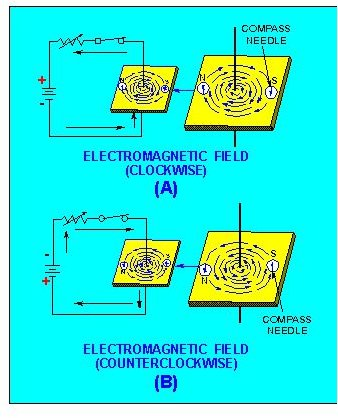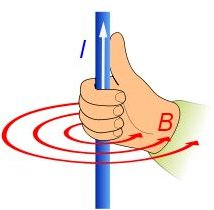# The Magnetic Effects of Electricity

In 1820, a Danish physicist named Hans Christian Oersted observed that the flow of a current through a wire causes the deflection of a nearby magnetic needle. This was a strong indication that the generation of a magnetic field is associated with the flow of electrons through a conductor.

## Magnetic Effect Of Electricity:

How to detect a magnetic field around a straight conductor carrying current:

A smooth sheet of cardboard with iron filings spread over is fixed in a horizontal plane with the help of a clamp. A straight wire passes through a hole made at the center of the cardboard.

A current passes through the wire when its two ends are connected to a battery. When the cardboard is gently tapped, we can observe that the iron filings arrange themselves along concentric circles. This clearly shows that a magnetic field is developed around the current carrying conductor.

To find the direction of the magnetic field, let us imagine a straight wire passing through the plane of the paper and perpendicular to it. A compass needle is then placed in such a way that its axis is always tangential to the plane of the circular magnetic field around the conductor. The compass is then left at rest.

When the current is inward, the direction of the magnetic field around the conductor is clockwise.

When the current direction is reversed – that is outward – the direction of the magnetic pole of the compass needle also changes showing the reversal of the direction of magnetic field. Now, the direction of the magnetic field is counterclockwise.

These facts prove that the direction of the magnetic field depends on the direction of the current in conductor. This is given by Maxwell’s rule.

## Magnetic Field around a Straight Conductor Carrying Current:## Right Hand Grip Rule## Law Supporting the Magnetic Effect of Electricity.

Maxwells’s Right Hand Corkscrew Rule:

If a right hand corkscrew is rotated along the direction of the current flow, then the direction of screw rotation gives the direction of the magnetic lines of force around the conductor. The direction of the magnetic field can also be determined by using the right hand grip rule (see image above).

Then comes the Biot-Savart Law:

Biot and Savart conducted many experiments to determine the factors that the generated magnetic field depends on.

The results of the experiments are summarized by their law (Biot-Savart Law).

Let us consider the current carrying conductor XY carrying current I. Consider a small element in the conductor AB=dI. P is a point at a distance r from a midpoint of AB.

According to the Biot and Savart Law, the Magnetic Induction dB at a point P due to the current flow through the element dI is:

1. Directly proportional to the current (I)
2. Directly proportional to the length of the element (dI)
3. Directly proportional to the sine of the angle between dl and the line joining element dI and the point P (sin Θ)
4. Inversely proportional to the square of the distance of the point P from the element dI (1/r2).Magnetic Induction dB (directly proportional) = ( I × dI × sinΘ ) / r2

Introducing the constant for Proportionality:

k = μ/ 4π

where k = constant of proportionality, μ = magnetic constant of the medium

So the magnetic induction produced by the small element dl is given by:

dB=μ/ 4π × ( I × dI × sinΘ ) / r2

This equation is the mathematical form of Biot-Savart Law and can measure the amount of magnetic induction dB produced due to the flow of current I through the conductor element dI.

References

Wikipedia Commons: Biot-Savart Law , Magnetic Field

Integrated Publishing: Electrical Engineer Training Series